Visualization of 3D incompressible flows

see  arXiv:1304.1884

Decompose a 3D divergence free velocity field into 3 components: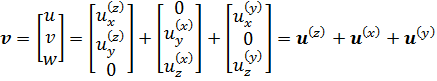So that two dimensional divergence of each component vanishes: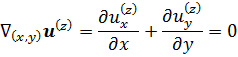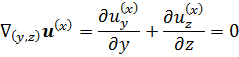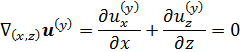Now, for each component define vector potential, which contains only one non-zero component: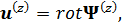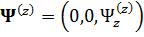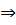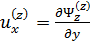,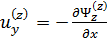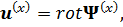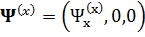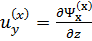,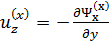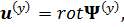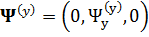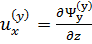,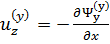Therefore, non-zero components of vector potentials are extensions of two-dimensional stream function: vectors of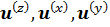are tangent to isosurfaces of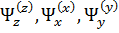, respectively.

Visualization of lid-driven flow in a cube

lid moves parallel to the diagonal, Re=1000: in (x,y,z) coordinates; main circulation perpendicular to diagonal plane

Visualization of natural convection in a laterally heated cube

Adiabatic horizontal and spanwise boundaries:  Ra=103      Ra=104      Ra=105      Ra=106      Ra=107      Ra=108

Conducting horizontal boundaries:  Gr=2.9×106

Animation of slightly supercritical oscillatory flows. Thermally conducting horizontal boundaries, Gr=3×106.

Multiple solutions for thermally insulated spanwise boundaries:  Regime 1,  Regime 2